# ⚡️ The computers are fast, but you don't know it

Humans have a shit sense of measurement, especially for quantities that they can't biologically perceive. For example, you intuitively know how much more heavy a 10kg object is than a 1kg object.

For such quantities, your sense of measurement can improve if you have some way to translate them into signals that the brain is familiar with. For example, have you seen these videos?

The second is my favorite. I eat one cup of rice every day. So I measure Jeff's wealth not just visually but also with my stomach.

Very recently, I did a few optimizations on a piece of code, which helped me intuitively understand how fast a computer can really go. Thought I'd share .

### What are we optimizing?

The function looks something like this:

```# note that this is a reduced form of the actual function
def aggregate(input_df):
weights = initialize_weights(len(input_df))

# input_df contains three columns timestamp, score & id
output_df = group_by_id(input_df)

# sorting happens within group
output_df = sort_by_time(output_df)
output_df = sort_by_score(output_df, desc=True)

# ranking happens within the group
output_df = rank_within_id(output_df)

output_df = multiply_weights(output_df, weights)
results = results_sum_in_each_group(output_df)

return results # is a list
```

This is a score aggregation function that's used by one of our Machine Learning (ML) services. This function is crucial to getting the model to work. Note that the output of this function needs to be computed in less than 500ms for it to even make it to production (where we need to do between 400-800 calls to this function under 500ms). I was asked to optimize it.

Okay let's measure how this function performs for a 1000 calls (assume each input will have 10 items max)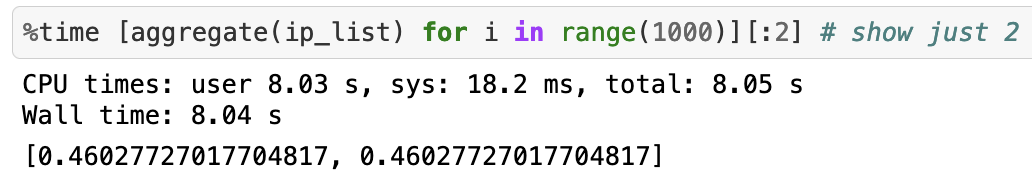Took ~8 seconds to do 1000 calls. Not good at all :( Well, I wanted to try for 1 million calls but it just takes over 20 minutes to do so. Let's see how we can do better:

### Optimization 1: Writing the algorithm without Pandas 🐼 + trivial algorithm improvements

Python's "Pandas" library is great for playing around with the data but it's horrible for production. If you find yourself using it in a production system then it's time to grow up . I replaced Pandas with simple python lists and implemented the algorithm manually to do the group-by and sort.

Also, the function for calculating weights was being re-initialized in every call, and all it did was initialize the same sequence of weights for some size of the array. This was a bonus and I pre-computed weights.

Here is how the function looked:

```WEIGHTS = initialize_weights(99999)
def aggregate_efficient(input_lists):
global WEIGHTS

# input_lists contain 2 lists
output_lists = algorithm_wizardry(input_lists)

output_lists = multiply_weights(output_lists, WEIGHTS)
results = results_sum_in_each_group(output_lists)

return results # is a list

aggregate_efficient(ip_list)
```

And this is how long it takes for 1 million calls: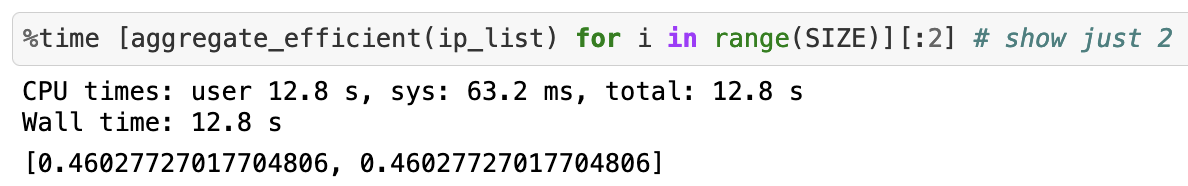So from 20 minutes, we have come down to 12 seconds! That's roughly a 9900% increase in speed.

This is enough for the function to go to production. But why stop here?

### Optimization 2: Cythonizing our functions

One of the simplest tricks to speed up a python function is to simply write it in Cython. Here is how to do it:

1. Create your `.pyx` function

The more `cdef` you can put here the better the optimization

```def aggregate_efficient_cyth(double[:] score_array,
double[:] time_array,
double[:] weights):

results = algorithm_wizardry(score_array,
time_array, weights)
return results
```

We add some compiler flags to make it go fast. Some say -O3 flag is dangerous but that's how we roll

```from distutils.core import setup
from Cython.Build import cythonize
from distutils.extension import Extension
from Cython.Distutils import build_ext

ext_modules = [
Extension("agg_cython",
["agg_cython.pyx"],
libraries=["m"],
extra_compile_args = ["-O3", "-ffast-math", "-march=native", "-fopenmp" ],
language="c++")
]
setup(name="agg_cyth_pure",cmdclass = {'build_ext': build_ext}, ext_modules=ext_modules,)
```

And this is how it performs for 1 million calls: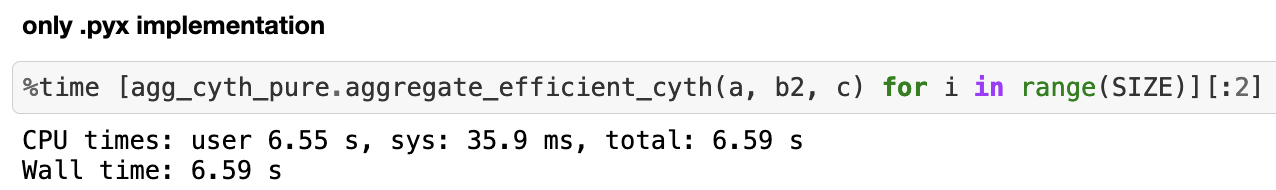`~6.59` seconds i.e. ~82% increase in the speed. We managed to almost half the time of the previous optimization :) This is awesome!

We will not stop here. Let's go nuts

### Optimization 3: Writing your function in pure C++

This is where the fun starts. This is one of the most important skills that I've been able to add to my tech inventory (thanks Rags ), and if performance really matters to you then this will help you:

1. Implement the function in pure C++
```#include "agg_cyth_fast.hpp"

using namespace std;

double agg_efficient(double score_array[],
long time_array[],
double weight_lookup[],
int N){

vector<double> results = algorithm_wizardry(score_array,
time_array, weight_lookup, N);
return results;
}
```
```#ifndef AGG_H
#define AGG_H

#include <iostream>
#include <map>
#include <vector>
#include <algorithm>

double agg_efficient(double[], long[], double[], int);
#endif
```
1. Write a `.pyx` file to talk to C++
```import numpy as np
from math import exp
from libc.math cimport exp as c_exp
from cython.parallel import prange
cimport cython

cdef extern from "agg_cyth_fast.hpp" nogil:
double agg_efficient(double[], long[], double[], int)

def agg_efficient_fs(double[:] score_array,
long[:] time_array,
double[:] weight_lookup):
cdef int N = len(time_array)
cdef double Y;
Y = agg_efficient(&score_array,
&time_array,
&weight_lookup, N);
return Y
```

Finally, we use a similar setup.py file as the previous optimization and build our function. This is effectively going to let us pass Python objects to a pure C++ function.

This is how it performs for 1 million calls: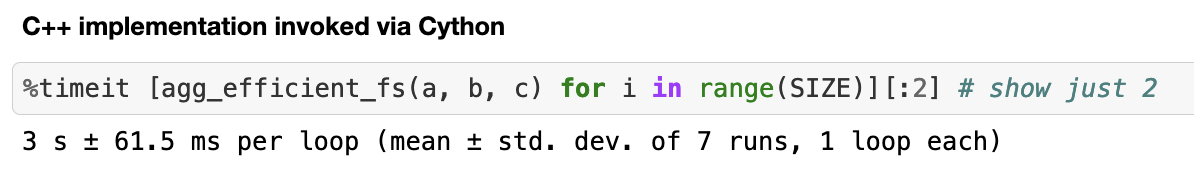It's crazy how fast pure C++ can be. We have sped up  the computation by ~119%! 🎉

We are not done yet. The .pyx file in this section has some code hidden but someone with a keen observation might have guessed the next optimization by looking at the imports.

### Optimization 4: Time to banish the Global Interpreter Lock (GIL)

Python has this annoying thing called GIL, which won't let your multi-threaded python code run faster than a single-threaded one.

If you really want to bully your computer, why do it on a single core?

We now, use prange to simply parallelize our computations.

Simply add this to the `.pyx` file:

```@cython.boundscheck(False)
@cython.wraparound(False)
def agg_efficient_fs_batch(double[:,:] score_array,
long[:,:] time_array,
double[:] weight_lookup):
cdef int M = len(score_array)
cdef double[:] Y = np.zeros(M)
cdef int i, N
for i in prange(M, nogil=True):
N = len(time_array[i])
Y[i] = agg_efficient(&score_array[i],
&time_array[i],
&weight_lookup, N);
return Y
```

This is how much it takes the same 1 million calls in parallel on a 4 core machine: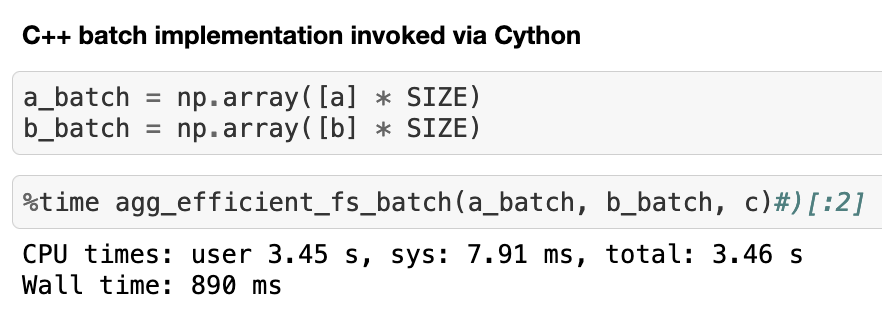That's an approximate ~237% increase in speed over the last optimization.

### To Summarize, for 1 million calls:

Optimization Time Taken Speedup Over Original
None 1200s -
Remove Pandas + Algo improvement 12s ~9900%
Cythonize Function 6.59s ~18109.4%
Pure C++ Implementation 3s ~33326.2%
Parallel C++ on 4 cores 890ms ~134731%
Parallel C++ on 32 cores 201ms ~596915%

I guess this is how fast computers can be